# Section 4.2: The Systematic Construction of Dynamic Models

## Section 4.2.1: Introduction

A complete discussion of how to develop chemical engineering models is really an complete course in chemical engineering, with practical experience besides! The objective of this section is thus only to show that there is a good, as opposed to a bad, way of constructing models. If this approach, which is systematic, is followed, then, assuming that the model builder's chemistry, physics and chemical engineering understanding of the process being modelled is correct, then there is a better chance than otherwise that the final model will be correct.

The basis of this approach to model building is that the equations which constitute a model are not arbitrary mathematical entities, but have a consistent physical basis. There are certain types of equation which describe different aspects of a model. A knowledge of this helps to ensure that all equations are written down.

Another point is that the set of model equations should be written without regard to how they might be solved. A confusing feature of some of the classical textbooks in chemical engineering is that the author, who knows the answer he wants to reach, slips steps in the solution process in amongst the construction of the model. The way to avoid confusion is to write all the equations down without attempting to perform any algebraic manipulation or simplification.

This section might therefore be subtitled: How to avoid algebra. The final models may be less elegant than those in some text books, but the computer will have no difficulty in solving them.

## Section 4.2.2: Procedure

The procedure which should be used to construct these models will now be introduced using a simple example of the flow into a tank.

## Step 1: Draw a Diagram

This is the first thing to do in attempting to model any system. Write down, conveniently on the diagram, but also separately with definitions, units etc., all the quantities which you can identify, assigning each an appropriate symbol. This helps to ensure that you identify and understand all the variables in the problem. Also identify any quantities which you expect to know or be able to choose, such as dimensions, physical properties or known feeds.

The figure below shows a tank whose outflow, through a pipe in the base of the tank, depends on the level in the tank. Two feeds are shown, but these are assumed to be of the same material.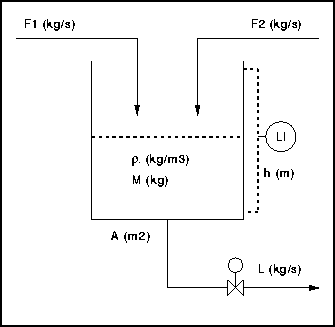Here we might identify the following initially.

Known quantities:

• F1, F2 - known mass flow rates (e.g. kg/s) into the tank
• A - tank cross section area, m2
•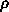- fluid density, kg/m3

Unknowns:

• M - mass holdup (e.g. kg) in the tank at any instant in time
• L - mass flowrate out of the tank
• h - level (e.g. m) in the tank

Other variables will be found to be necessary as we proceed with building the model. These can just be added to the list.

## Step 2: Material and Energy Balance Equations

Balance equations are the most fundamental type of equation, and have the advantage that we know exactly how many there must be in any problem. About every element which we model, there will be one material balance for each chemical species of interest present, and, if temperature effects are being modelled, one energy balance.

In this case we have one species and no temperature effects. We are modelling a single element, namely a tank, and so there is one balance equation.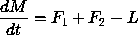Note the following.

• This is an unsteady state or dynamic (i.e. time varying) system, so the balance equations are ordinary differential equations.
• These must have the form: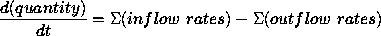• In this equation quantity must be an extensive quantity, e.g. kg, moles, Joules etc. Do not try to write so-called material balance equations involving temperatures or concentrations.
• Flowrates must similarly be kg/s, kmol/hr, Watts, etc.

## Step 3: Rate Equations

The flowrates on the r.h.s. of the material balances are typically determined by rate equations which relate them to some driving force or potential variables. Here for example: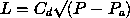This says that the outflow rate depends on the pressure P at the base of the tank, the downstream pressure Pa and a discharge coefficient.

There must be a rate equation for every unknown material or energy flowrate which appears in a balance equation.

In the above equation there are some new variables to add to the list. Cd and Pa are typically known quantities. P is an additional unknown. It is an intensive quantity in the thermodynamic sense and physically represents a driving force which can cause an extensive quantity, e.g. mass, to flow. Other driving force variables are temperature (heat) and concentration (flow or reaction of a chemical species).

The general form of a rate equation is usually: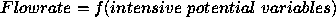## Step 4: Equations of State

In this context we give this name to the class of equations which relate intensive thermodynamic quantities to each other and to extensive quantities. A classic equation of state (e.o.s.) is the ideal gas equation, which does not however apply here. It relates intensive temperature and pressure and extensive molar amount.

We look here for an e.o.s. which relates the intensive potential variable P introduced above to an extensive holdup variable, or more generally, a relationship amongst several such variables.

Note first that: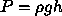And also that: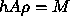These equations together provide a relationship between the intensive driving force variable P and the extensive mass holdup M.

Equations of state are sometimes not entirely obvious to identify, and they sometimes, as here, introduce further variables. Their general form is: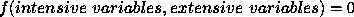If h andhad not been identified as variables of interest we might have here gone straight to a single e.o.s., namely: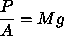## Step 5: Count the Equations and Unknowns

If the model has been correctly formulated then there will be the same number of equations and unknowns.

There are 4 equations above. The unknowns in summary are M, h, L, P. Hence the model appears to be correct.

## Summary

Notice that there are three categories of equation:

• Balance
• Rate
• EOS

There are also three kinds of variable:

• Extensive holdups
• Extensive rates
• Intensive potentials

The balance equations involve holdups and rates, the rate equations rates and potentials, and the e.o.s. potentials and holdups. This establishes a circular relationship as shown in the figure.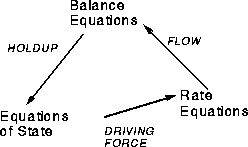## Section 4.2.3 Example 2: Fed Batch Reactor

The second order reaction: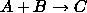is carried out isothermally in a tank which is initially filled with B, and to which A is gradually added as the reaction proceeds. When the reaction is sufficiently complete, the product is run off. The feed rate fA kmol/hr of A is a known function of time. The emptying process is not to be modelled.

## Balance Equations

There is one for each component. These will be written in molar quantities.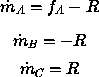Here the m are the mass in the tank in kmol and R is the rate of reaction in kmol/hr.

## Rate equation

At this stage we have to define the rate R:

R = k CA CB V

Here V is reactor volume and k a specific rate constant in suitable units.

Since the feed rate of A is not an unknown there is only one rate equation.

There are thus, so far, 4 equations in 7 unknowns: mA, mB, mC, R, CA, CB and V

## Equations of State

At least 3 further equations are required. It is clear that the concentrations and molar holdups are related, so that:
•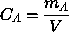•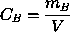It is less obvious that an equation can be written for V in terms of the molar densities of the species, which are assumed to be known: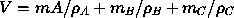This completes the model.

## Section 4.2.4 Example 3: Sparged Steam Water Heater

A supply of hot water at temperature T is required at a rate W (kg/s) and this is to be provided by sparging steam at temperature Ts at a rate S (kg/s) through a mass M kg of water in a tank. The tank is fed with cold water at temperature Tc.

As before the first thing to do is to draw a diagram of the process as shown below.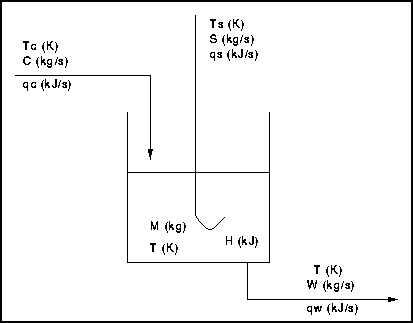Known Quantities : M, Tc, Ts, W, S

Unknown Quantities : C, H, T, qc, qs, qw

The objective of the exercise is to obtain equations so that all the above quantities can be calculated.

## Balance Equations

The material balance can be written formally as: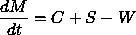(It is, however, intended that the amount M of water in the tank shall be constant, i.e.: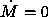So in this case the material balance reduces to an algebraic equation from which the required cold water feed rate C kg/s can be determined.

C = W - S

This does not affect the model building process, although it will affect any solution method.)

There is a single energy balance differential equation: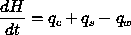Here H, in Joules, is the energy holdup in the vessel and the q are energy flowrates (e.g. in W) associated with the various water and steam streams.

## Rate Equations

These are somewhat different fron their classical form, but the following can be thought of as defining each of the three q variables above.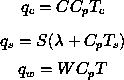Cp is taken as an average heat capacity,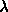as a known constant latent heat and the implied enthalpy reference temperature is zero on the temperature scale used.

## Equations of state

Since it is not always immediately obvious what e.o.s. are required, it is useful at this point to count up the equations and unknowns so far identified as a guide to the number of equations of state required. Note that this will indicate the minimum number of equations required, since when these are written it may be found that they introduce further unknowns.

In the 5 equations above the 6 unknowns are : C, H, qc, qs, qw, T

One further equation is needed.

The temperature in the tank, T, is related to the total enthalphy by the equation of state:

H = M Cp T

We thus have a complete model with 6 equations and unknowns.